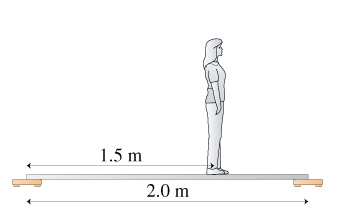# Problem: Suppose the woman in the figure is 54 kg, and the board she is standing on has a 10kg mass. What is the reading on each of the scales?

###### FREE Expert Solution

In this problem, we consider a situation of equilibrium.

When the system is at equilibrium, the sum of the clockwise moments is equal to the sum of counterclockwise moments.

We can then calculate the normal components as follows:

The board is of mass 10kg and the woman i of mass 54 kg.

86% (308 ratings)###### Problem DetailsSuppose the woman in the figure is 54 kg, and the board she is standing on has a 10kg mass. What is the reading on each of the scales?